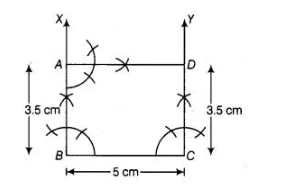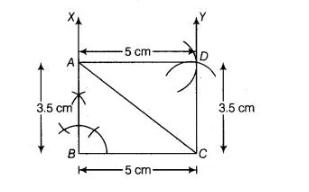# Construct a rectangle whose adjacent`
Question:

Construct a rectangle whose adjacent sides are of lengths 5 cm and 3.5 cm.

Solution:

We know that, each angle of a rectangle is right angle (i.e., 90°) and its opposite sides are equal and parallel.
To construct a rectangle whose adjacent sides are of lengths 5 cm and 3.5 cm, use the 1 following steps

1. Draw a line segment BC of length 5 cm.
2. Now, generate an angle of 90° at points B and C of the line segment BC and plot the parallel lines BX and CY at these points.1. Cut AB and CD of length 3.5 cm from BX and CY, respectively.
2. Draw an angle 90° at one of the point A or D and join both points by a line segment AD of length 5 cm.
Thus, ABCD is the required rectangle with
adjacent sides of length 5 cm and 3.5 cm.

Alternate Method
To construct a rectangle ABCD whose adjacent sides are of lengths 5 cm and 3.5 cm, use the following steps

1. Draw a line segment $S C$ of length $5 \mathrm{~cm}$.

2. Now, draw an $\angle X B C=90^{\circ}$ at point $B$ of line segment $S C$.

3. Cut a line segment $A B=3.5 \mathrm{~cm}$ from the ray $B X$ and join $A C$.1. Now, from A, point D is at a distance of 5 cm. So, having A as centre draw an arc of radius 5 cm.
2. From C, point D is at a distance of 3.5 cm. So, having C as centre draw an arc of radius 3.5 cm which intersect previous arc (obtained in step iv) at D.